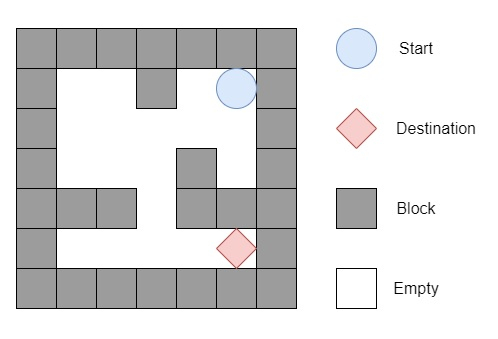# The Maze in C++

Suppose there is a ball in a maze with empty spaces and walls. Now the ball can go through empty paths by rolling any direction like up, down, left or right, but it won't stop rolling until hitting a wall. When the ball stops, it could choose the next direction.

We have to start position of ball, the destination and the maze, we have to check whether the ball could stop at the destination. The maze is represented by one 2D array. Here 1 indicates the wall and 0 indicates the empty space. The borders of the maze are all walls. The start and destination coordinates are represented by row and column indices.

So, if the input is like a maze represented by a 2D array

 0 0 1 0 0 0 0 0 0 0 0 0 0 1 0 1 1 0 1 1 0 0 0 0 0

start position is (0, 4) destination position is (4, 4), then the output will be true, One possible way is − left to down to right.To solve this, we will follow these steps −

## Example

Let us see the following implementation to get a better understanding −

Live Demo

#include <bits/stdc++.h>
using namespace std;
class Solution {
public:
bool hasPath(vector<vector<int<>& grid, vector<int<& start, vector<int<& destination) {
int n = grid.size();
int m = grid.size();
queue<vector<int< > q;
q.push(start);
set<vector<int< > visited;
visited.insert(start);
while (!q.empty()) {
vector<int< curr = q.front();
q.pop();
int x = curr;
int y = curr;
if (destination == x && destination == y)
return true;
int i = x;
while (i + 1 < n && !grid[i + 1][y])
i++;
if (!visited.count({ i, y })) {
visited.insert({ i, y });
q.push({ i, y });
}
i = x;
while (i - 1 >= 0 && !grid[i - 1][y])
i--;
if (!visited.count({ i, y })) {
visited.insert({ i, y });
q.push({ i, y });
}
i = y;
while (i + 1 < m && !grid[x][i + 1])
i++;
if (!visited.count({ x, i })) {
visited.insert({ x, i });
q.push({ x, i });
}
i = y;
while (i - 1 >= 0 && !grid[x][i - 1])
i--;
if (!visited.count({ x, i })) {
visited.insert({ x, i });
q.push({ x, i });
}
}
return false;
}
};
main(){
Solution ob;
vector<vector<int<> v = {{0,0,1,0,0},{0,0,0,0,0},{0,0,0,1,0},{1,1,0,1,1},{0,0,0,0,0}};
vector<int< v1 = {0,4}, v2 = {4,4};
cout << (ob.hasPath(v, v1, v2));
}

## Input

{{0,0,1,0,0},{0,0,0,0,0},{0,0,0,1,0},{1,1,0,1,1},{0,0,0,0,0}},{0,4},{4,4}

## Output

1